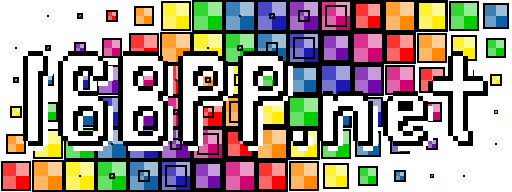# Random Art in WebGL

K=

Please read this page here if you're new to random art. This toy differs from that description, in that `(const)`, doesn't use 4 channels (ARGB) but only three (RGB). E.g. `(const 1 0.75 -0.4 0.9)` is not valid but `(const 0.75 -0.4 0.9)` is. And compared to the original Python implementation `(mix)`'s weight parameter (the first one) does work.

If you don't what to do, I suggest setting `K` to somewhere between 2 and 5, and pressing the "Generate Random" button a few time; You'll start to spot the patterns of how the language works.

Grammar:

Numbers:
`R, G, B``[-1, 1]`
`f`[any valid real number]

Terminals:
N`(const R G B)`
N`(var x)`
N`(var y)`

Non-Terminals:
N`(sum N N)`
N`(mul N N)`
N`(mod N N)`
N`(sin f f N)`
N`(level f N N N)`
N`(mix N N N)`
N`(tent N)`
N`(well N)`